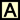Parabolas
 Home > Lessons > Conic Sections > Parabolas Search | Updated April 9th, 2022
Introduction

In this section, you will learn how to graph parabolas. Here is a list of the sections within this webpage:

 How to Graph a Parabola The definition of a parabola is the set of all points that are equidistant to a focal point and a line called a directrix. Howver, it is best recognized by its classic u-shape.      Parabolas either open up, down, right, or left. The graphic below will show you how a parabola looks in comparison to its equation.When the x-value is being squared, the parabola opens up when the p-value is positive and down when the p-value is negative.      On the other hand, when the y-value is being squared, the parabola opens right when the p-value is positive and left when the p-value is negative.      The h-value and the k-value represents the location of the vertex, V(h,k). Therefore, it is important to know the location of the vertex in order to determine the exact equation of a parabola. Conversely, when the equation of a parabola is known, the exact location of the vertex can be determined.      Here is a video and a quiz that will help you understand and assess your understanding of parabolas.ideo: Parabolas: Directrix, Focus, Vertexideo: Parabolas: Graphingideo: Parabolas: Building Equationsuiz: Parabolasuiz: Paraboloid Receiver
 Videos Here are videos related to the lessons above.ideo: Parabolas: Directrix, Focus, Vertexideo: Parabolas: Graphingideo: Parabolas: Building Equations
 Quizmasters After reading the lessons, try our quizmasters. MATHguide has developed numerous testing and checking programs to solidify these skills:uiz: Parabolasuiz: Paraboloid Receiver Activities Here are activities related to the lessons above.ctivity: Conics: Supplemental Related Lessons and Quizmasters Here related lessons and quizmasters.esson: Circlesesson: Ellipsesesson: Hyperbolasesson: Conic Sectionsesson: Graphing Linesesson: The Point-Slope Formulaesson: Graphing Linear Inequalitiesesson: Flight of a Projectileesson: Completing the Squareuiz: Parabolic Functions